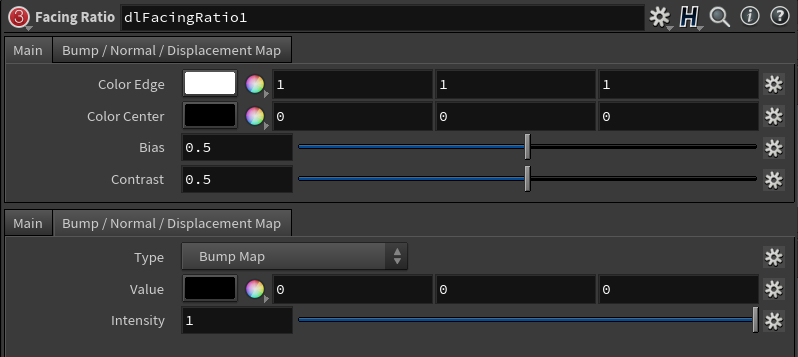##### Page tree
Go to start of banner

# Facing Ratio→ Edge Colour

The colour seen at grazing angles (on the edge of the objects).

→ Center Colour

The colour seen at the center of the colour, where the normal is usually more facing the viewer.

→ Bias

This is a value in the [ 0..1 ] range used in a Bias Function to affect how the output is interpolated between the center and the edge. A bias function is similar to a Gamma function but with the range set between 0 and 1.  A value of 0.5 interpolates linearly between center and edge.→ Contrast

Applies a contrast to the output.

→ Normal

The normal to use then computing the facing ratio. If nothing is connected to this plug, the surface normal will be used.

• No labels
Write a comment…